# Working with Built-In Objects

 [ LiB ]

### Working with Built-In Objects

JavaScript and JScript provide access to a collection of built-in objects. These objects are always available regardless of the environment in which your scripts execute. These objects include the Array, Boolean, Date, Function, Math, Number, Object, and String objects.

NOTE

Notice that built-in object names are always capitalized.

#### The Array Object

I touched on the Array object earlier this morning. To work with arrays, you must create an instance of an Array object.

An array is an indexed list of values that can be referred to as a unit. You can work with array elements by referencing their names and index numbers . An array has a length property that tells you how large the array is, as well as an assortment of methods for manipulating its contents. These include methods to sort an array, to concatenate two arrays into a single array, to add or remove elements from the array, and to reverse the order of the array. The first entry in an array is assigned the index 0.

One thing I did not show you earlier is how to create multidimensional arrays; I showed you only single-dimension arrays. An example of a single-dimension array is a list of automobiles. For example, the following list contains three elements and is a single-dimension array:

• Ford

• Honda

• Jeep

A multiple-dimension array can be used to represent data stored in table format. For example, the following information could be stored in a two-dimensional array:

 Ford Red \$4,000 Honda Blue \$4,888 Jeep Green \$12,500

The following script demonstrates how to build and display the contents of a multidimensional array:

` <HTML>   <HEAD>     <TITLE>Script 2.27 - Creating a Multi-Dimensional Array</TITLE>   </HEAD>   <BODY>     <SCRIPT LANGUAGE="JavaScript" TYPE="Text/JavaScript">     <!--Start hiding JavaScript statements       var arraySize       //Part 1 - Prompt user for array size       arraySize = window.prompt("How many cars do you plan to list?","");        MyArray = new Array(arraySize);       //Part 2 - Populate the array with user supplied information       for (row=0; row < arraySize; row++ ) {         MyArray[row] = new Array(3);         MyArray[row] = window.prompt("Type of car?","");         MyArray[row] = window.prompt("Color of car?","");         MyArray[row] = window.prompt("Sale price?","");       }       //Part 3 - Display the contents of the array       for (row=0; row < arraySize; row++ ) {         for (column=0; column < 3; column++ ) {           document.write(MyArray[row][column] + " ");         }         document.write("<BR>");       }     // End hiding JavaScript statements -->     </SCRIPT>   </BODY> </HTML> `

I've used comments to divide this script into three parts , each of which performs a specific activity. In Part 1, I defined a variable called arraySize that contains the number of cars the user wants to enter. I then created an array called MyArray using the values contained in arraySize . If you think of a multidimensional array as a table, then this step defines the table length.

In Part 2, I added a for loop that creates the second dimension of the array. In this case, the for loop starts at 0, incrementing by 1 until it has executed for each element in the array. Again, if you look at the multidimensional array as a table, you can see that this step populates each column in the table as it iterates from top to bottom on a row-by-row basis. In each iteration, the user is asked to enter the type, color, and price of a car. In its first iteration, the script starts at row 0 (MyArray) and populates each column in row 0 (MyArray, MyArray, MyArray) . On its next iteration, the value of row has been incremented by 1 to 1. Again, the script prompts the user for information about the car and populates the cells in the second row (MyArray, MyArray, MyArray) . The process repeats as many times as the user has cars to enter.

The result is a multidimensional array that can be represented as a table. In this example, the table is three rows and three columns deep and can be mapped out as shown in Figure 2.18. As Figure 2.19 shows, individuals cells can be referenced.

##### Figure 2.18. Building a two-dimensional table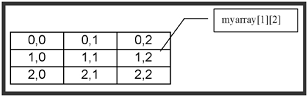##### Figure 2.19. A coordinate map of the two-dimensional table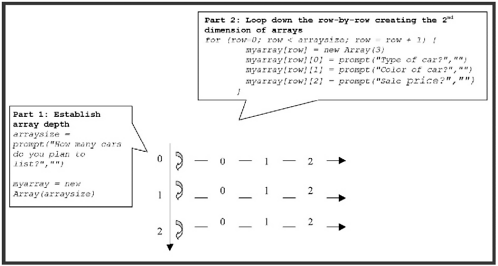In Part 3, the script prints out the contents of the multidimensional array using nested for loops . The first loop controls the row of the table that is processed. It starts at array element 0 and increments by 1 until the entire array has been processed . The second loop prints the contents of the multidimensional array by executing repeatedly for each row in the table, starting at column 0 and finishing with column 2.

Figure 2.20 shows the results I received when I tested the script.

##### Figure 2.20. An example of a multidimensional array#### The Boolean Object

JavaScript and JScript allow you to treat Boolean values like objects. You need to use the following syntax to create new Boolean objects.

`  BooleanObject  = new Boolean(  value  ); `

In this syntax, value must be either true or false . If you assign a Boolean object a value other than true or false , the value will be converted to a Boolean value. If a value of NaN , null, undefined, -0, or 0 is found, then the values assigned will be false ; otherwise the value is converted to true .

Following is a list of sample Boolean objects:

` TestObject1 = new Boolean();        //initial value is false TestObject2 = new Boolean(-0);      //initial value is false TestObject3 = new Boolean(false);   //initial value is false TestObject4 = new Boolean(true);    //initial value is true  TestObject5 = new Boolean("Hello"); //initial value is true TestObject6 = new Boolean("false"); //initial value is true `

NOTE

There is a distinction between false and "false" in the last two examples.This is because "false" is a string, and false is a keyword.

By using Boolean objects instead of simple Boolean values, you can take advantage of three Boolean object methods:

• toSource(). Used internally to return a string that represents the Boolean object's source code.

• toString(). Returns a string that represents the object.

• valueOf(). Gets the object's value.

Each of these methods is demonstrated in the following example:

` <HTML>   <HEAD>     <TITLE>Script 2.28 - Working with the Boolean Object</TITLE>   </HEAD>   <BODY>     <SCRIPT LANGUAGE="JavaScript" TYPE="Text/JavaScript">     <!--Start hiding JavaScript statements       TestObject = new Boolean(false);    //initial value is false       document.write(TestObject.toString() + "<BR>");       document.write(TestObject.valueOf());     // End hiding JavaScript statements -->     </SCRIPT>   </BODY> </HTML> `

#### The Date Object

JavaScript and JScript do not provide a date value. Instead, you must use the Date object to insert dates and times in your scripts. The Date object does not have any properties but does provide access to a large number of built-in methods.

Date information is based on the number of millions of seconds that have passed since January 1, 1970. You create instances of Date objects using the new operator as demonstrated below.

` Today = new Date(); document.write(today); `

This example creates a variable called today and sets it equal to the current date. The second line displays the result of the today variable. If you were to put these statements into a script and run it, you'd get a result similar to the following when processed by Netscape Communicator:

` Thu Dec 10 12:21:29 GMT-0500 (Eastern Standard Time) 2000 `

As you can see, the default format for the Date object is the day of the week followed by the name of the current month, its numeric date, the current time, the offset from GMT time, time-zone information, and finally the current year.

If this is a bit more information than you want or need, you can use various Date methods to retrieve more specific information. The following example demonstrates some of the more commonly used Date methods. In this script, the result of each method is assigned to an array element in an array named MyDate , the total length of which is eight elements (MyDate[x] = "message = " + TodaysDate.datemethod()) . After executing the Date object's methods and loading the array, the script executes a for loop to spin through the array and print its contents. The loop begins with array element 0 (i = 0) and increments by 1 (i = i + 1) with each iteration until it reaches the end of the array (that is, until i < arrayLength is no longer true ).

` <HTML>   <HEAD>      <TITLE>Script 2.29 - Using the Date Object</TITLE>   </HEAD>   <BODY>     <SCRIPT LANGUAGE="JavaScript" TYPE="Text/JavaScript">     <!-- Start hiding JavaScript statements       var arrayLength;       TodaysDate = new Date();       document.write("<B>Today's date is: </B>" + TodaysDate + "<P>");       MyDate = new Array(8);       MyDate = "thedate = "   + TodaysDate.getDate();       MyDate = "theday = "    + TodaysDate.getDay();       MyDate = "theyear = "   + TodaysDate.getFullYear();       MyDate = "thehour = "   + TodaysDate.getHours();       MyDate = "theminute = " + TodaysDate.getMinutes();       MyDate = "themonth = "  + TodaysDate.getMonth();       MyDate = "thesecond = " + TodaysDate.getSeconds();       MyDate = "thetime = "   + TodaysDate.getTime();       arrayLength = MyDate.length;       document.write("<B>Array of Date Method Examples</B> <BR>");       for (var i = 0; i < arrayLength; i++ ) {         document.write(MyDate[i], "<BR>");       }      // End hiding JavaScript statements -->     </SCRIPT>   </BODY> </HTML> `

Figure 2.21 shows the result of executing this script.

##### Figure 2.21. Using an array to store and display the results of data gathered using the Date object's methods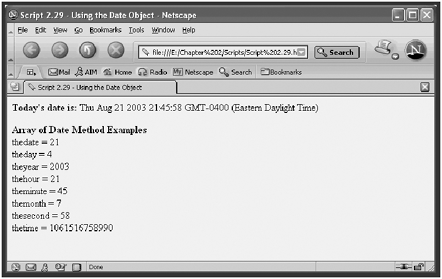NOTE

When working with the Date object's methods, you must use 0 to 59 when representing minutes and seconds, 0 to 23 to represent hours, 0 to 6 to represent days, 1 to 31 when working with dates, and 0 to 11 to represent months. In the example, the date given is Friday, February 23, 1996. Note, however, that the getMonth() method returns 1, not 2, for February as you'd expect. Remember that computer logic makes January month 0, February month 1, and so on.

The Date object also provides a set of methods for setting the value of Date objects, including:

• setDate(12)

• setFullYear(2000)

• setHours(11)

• setMinutes(30)

• setMonth(4)

• setYear(2000)

#### The Function Object

JavaScript and JScript also enable you to create Function objects and provide you with access to Function properties and methods that are not available to normal functions. Function objects are easier to work with when your functions are composed of a single line of code. However, because of inefficiencies inherent in Function objects you are better off sticking with declared functions. The following example outlines the syntax that you must follow when using the Function object.

`  FunctionName  = new Function([  p1,.......pn  ]  body  ) `

In this syntax, p1 through pn are arguments that Function objects receive when called, and body is the script statement that is compiled as the function.

For example, the following code creates a Function object named Warn :

` Warn = new Function("alert('HI!')"); `

You can call this function as follows :

` Warn(); `

The preceding Function object is equivalent to the following declared function:

` function Warn() {   window.alert("Warning: You should not run this script!");   return; } `

NOTE

Because the Function object is compiled, it is less efficient than using declared functions. Therefore, I recommend that you avoid using compiled functions and the Function object.

The following script demonstrates the use of the previous Function object examples.

` <HTML>   <HEAD>     <TITLE>Script 2.30 - Working with the Function Object</TITLE>   </HEAD>   <BODY>     <SCRIPT LANGUAGE="JavaScript" TYPE="Text/JavaScript">     <!--Start hiding JavaScript statements       Warn = new Function("window.alert('Warning: You should not run this script!')");       Warn();     // End hiding JavaScript statements -->     </SCRIPT>   </BODY> </HTML> `

##### Figure 2.22. Using the Function object in place of regular functions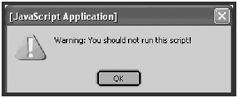#### The Math Object

The Math object provides mathematical constants in the form of properties. It also provides mathematical functions in the form of methods. Earlier this morning, I demonstrated several Math properties and methods. Tables 2.5 and 2.6 provide a review of the Math object's major properties and methods.

##### Table 2.5. SUMMARY OF MATH PROPERTIES

Property

Description

E

Euler's constant (2.718)

LN2

Natural logarithm of 2 (2.302)

LN10

Natural logarithm of 10 (.693)

LOG2E

Base 2 logarithm of e (.434)

LOG10

Base 10 logarithm of 10 (1.442)

PI

Ratio of the circumference of a circle to its diameter (3.141549)

SQRT1_2

Square root of ? (.707)

SQRT2

Square root of 2 (1.414)

##### Table 2.6. SUMMARY OF MATH METHODS

Method

Description

abs()

Returns the absolute value

cos(), sin(), tan()

Trigonometric functions

acos(), asin(), atan()

Inverse trigonometric functions

exp(), log()

Exponential and natural logarithms

ceil()

Returns the lowest integer greater than or equal to the argument

floor()

Returns the highest integer less than or equal to the argument

min(x,y)

Returns either x or y , depending on which is lower

max(x,y)

Returns either x or y , depending on which is higher

pow(x,y)

Returns the value of x y

random()

Returns a random number between 0 and 1

round()

Rounds the argument to the nearest integer

sqrt()

Returns the square of the argument

#### The Number Object

The Number object lets you treat numbers like objects and store numerical constants as properties. The values of these constants cannot be changed. You will most likely never need to know or work with the Number object, but just in case, Table 2.7 outlines its properties.

##### Table 2.7. SUMMARY OF THE NUMBER OBJECT'S PROPERTIES

Property

Description

MAX_VALUE

Largest possible number

MIN_VALUE

Smallest possible number

NaN

Not a number

NEGATIVE_INFINITY

Positive infinity

POSITIVE_INFINITY

Negative infinity

You define an instance of the Number object using the new keyword as demonstrated below.

` MyNumber = new Number(99.99); `

#### The Object Object

This is the object on which all other objects are based. This means that all objects inherit this object's properties and methods.

You can create new Object objects by specifying an Array, Boolean, Function, Number , or String object in the Object() constructor as shown here:

` MyNumberObject = new Object(99.99); MyStringObject = new Object("Testing"); `

NOTE

A constructor is a function that each object provides that you can use to create instances of objects. For example, to create an instance of a String object named MyString , you would specify the following MyString = new String("Add your message text here.") .

You'll probably never see anybody create objects in this manner. Instead, you'll see objects created using constructors specific to each object type, such as these constructors:

• Array()

• Boolean()

• Function()

• Number()

• String()

#### The String Object

As you have already learned, the String object enables you to work with strings as objects. However, you shouldn't need to use this object because JavaScript and JScript automatically converts simple strings into temporary String objects whenever you use a String object method or property on them.

The String object has just one property, length , which contains the number of characters that comprise the String object. However, the String object has a number of useful methods for manipulating the String object, as outlined in Table 2.8.

##### Table 2.8. COMMLY USED STRING OBJECT METHODS

Method

Description

charAt()

Returns the character at the specified position in the string where the index of a String object begins at zero

concat()

Combines two strings into one new string

fromCharCode()

Creates a string value based on the supplied code set

indexOf()

Returns the position of a specified substring

lastIndexOf()

Returns the last position of a specified substring

slice()

Creates a new string using a portion of the current string

split()

Organizes a string into an array

substring()

Returns the specified portion of a string

toLowerCase()

Returns the string in all lowercase characters

toUppercase()

Returns the string in all uppercase characters

The following example creates two String objects and then demonstrates the use of various String methods using these String objects and displays their results with the document.write() method.

` <HTML>   <HEAD>     <TITLE>Script 2.31 - Working with the String Object</TITLE>   </HEAD>   <BODY>     <SCRIPT LANGUAGE="JavaScript" TYPE="Text/JavaScript">     <!-- Start hiding JavaScript statements       MyString1 = new String(" He is gone now!");       MyString2 = new String("Once upon a time there was a little boy " +         "who cried wolf.");       document.write("<B>MyString1 - </B>" + MyString1 + "<BR>");       document.write("<B>MyString2 - </B>" + MyString2 + "<BR>");       document.write("<B>MyString2.charAt(5) - </B>" + MyString2.charAt(5) +          "<BR>");       document.write("<B>MyString2.charCodeAt(5) - </B>" +         MyString2.charCodeAt(5) + "<BR>");       document.write("<B>MyString2.indexOf('little') - </B>" +         MyString2.indexOf("little") + "<BR>");       document.write("<B>MyString2.lastIndexOf('who') - </B>" +         MyString2.lastIndexOf("who") + "<BR>");        document.write("<B>MyString2.substring(15) - </B>" +         MyString2.substring(15) + "<BR>");       document.write("<B>MyString2.substring(15,22) - </B>" +         MyString2.substring(15,22) + "<BR>");       document.write("<B>MyString2.toUpperCase() - </B>" +         MyString2.toUpperCase() + "<BR>");       document.write("<B>MyString2.toLowerCase() - </B>" +         MyString2.toLowerCase() +"<BR>");       document.write("<B>MyString2.concat(MyString1) - </B>" +         MyString2.concat(MyString1) + "<BR>");       document.write("<B>MyString2.slice(' ') - </B>" +         MyString2.slice(" ") + "<BR>");     // End hiding JavaScript statements -->     </SCRIPT>   </BODY> </HTML> `

Figure 2.23 shows the result of loading the page created in this example.

##### Figure 2.23. A demonstration of String object methods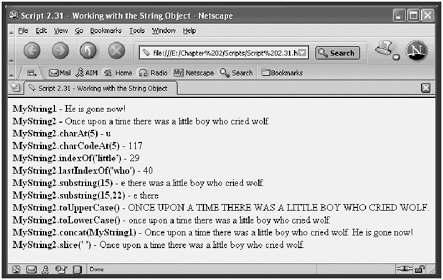[ LiB ]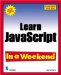Learn JavaScript In a Weekend, Second Edition
ISBN: 159200086X
EAN: 2147483647
Year: 2003
Pages: 84